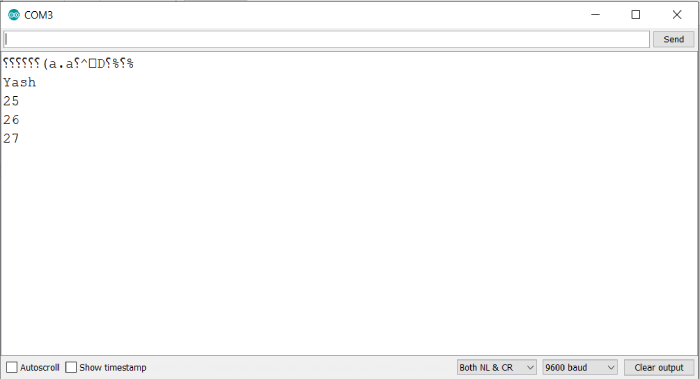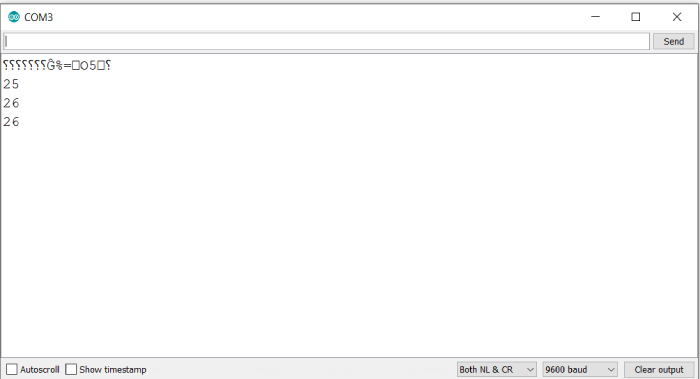# Structs in Arduino program

A struct is simply a collection of different types of variable. Structs in Arduino mimic the structs in C language. So, if you are familiar with C structs, Arduino structs shouldn’t be an issue. The struct declaration syntax is as follows −

Syntax

struct structName{
item1_type item1_name;
item2_type item2_name;
.
.
.
itemN_type itemN_name;
}

An example is given below −

Example

struct student{
String name;
int age;
int roll_no;
}

The elements of a struct are accessed using the . (dot) notation. This notation can be used for both reading the elements of a struct, or changing them.

## Example

The following example illustrates this −

struct student{
String name;
int age;
int roll_no;
};

void setup() {
// put your setup code here, to run once:
Serial.begin(9600);
Serial.println();
student A = {"Yash", 25, 26};
Serial.println(A.name);
Serial.println(A.age);
Serial.println(A.roll_no);

A.age = 27;
Serial.println(A.age);
}

void loop() {
// put your main code here, to run repeatedly:
}

## Output

The Serial Monitor output is shown below −As you can see, we were able to both read the elements and change an element of the struct (age), using the dot notation.

Note that while the declaration of the struct contains a semi-colon between each variable, the creation of the struct contains a comma −

student A = {"Yash", 25, 26};

Once a struct has been created, you can treat it like any other data type. You can even have functions returning the struct.

For example −

student funcA(){
student A = {"Yash", 25, 26};
return A;
}

In some literature related to structs, you will come across the → notation. This is used in place of dot notation when you are dealing with a pointer to the struct.

## Example

The following example demonstrates that.

struct student{
String name;
int age;
int roll_no;
};

student increaseAge(student *B){
B→age = B→age + 1;
return *B;
}

void setup() {
// put your setup code here, to run once:
Serial.begin(9600);
Serial.println();

student A = {"Yash", 25, 26};
Serial.println(A.age);
student *C = &A;

student D = increaseAge(C);
Serial.println(A.age);
Serial.println(D.age);

}
void loop() {
// put your main code here, to run repeatedly:
}

## Output

The Serial Monitor output is −As you can see, the struct pointer C was pointing to A. When the age was incremented in the increaseAge function, it reflected in the struct A as well.